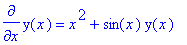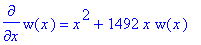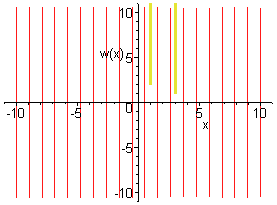f205hw1s.mws

B. Dodson/Sect. 12

> with(DEtools):

> diff(y(x),x)=x^2+sin(x)*y(x);> diff(w(x),x)=x^2+1492*x*w(x);> DEplot(diff(w(x),x)=x^2+1492*x*w(x),w(x),x=-10..10,{[1,2],[3,1]},w=-10..10,arrows=line);> DEplot(diff(w(x),x)=x^2+42*x*w(x),w(x),x=-10..10,{[1,2],[3,1]},w=-10..10,arrows=line);

> DEplot(diff(w(x),x)=x^2+2*x*w(x),w(x),x=-10..10,{[1,2],[3,1]},w=-10..10,arrows=line);

This DE is w' = x^2 + 2xw --- it is the linear DE w' -2xw = x^2 --- looks

like the blackboard example was also linear. We have P = -2x here, while

the example there had P = -sin(x). Let's try a smaller P to get smaller values

on the slope field (which will be more visible in the plot of the slope field).

>

> DEplot(diff(w(x),x)=x^2+2*x*(1+x^2)*w(x),w(x),x=-1.6..3.2,{[1,2],[3,1]},w=-5..5,arrows=line);>